Next: Magnetic Moments Up: Spin Angular Momentum Previous: Wavefunction of Spin One-Half

# Rotation Operators in Spin Space

Let us, for the moment, forget about the spatial position of the particle, and concentrate on its spin state. A general spin stateis represented by the ket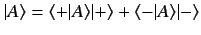(439)

in spin space. In Section 4.3, we were able to construct an operator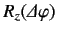that rotates the system through an angle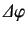about the-axis in position space. Can we also construct an operator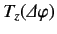that rotates the system through an angleabout the-axis in spin space? By analogy with Equation (351), we would expect such an operator to take the form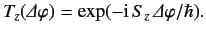(440)

Thus, after rotation, the ketbecomes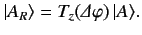(441)

To demonstrate that the operator (440) really does rotate the spin of the system, let us consider its effect on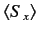. Under rotation, this expectation value changes as follows: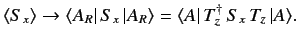(442)

Thus, we need to compute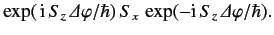(443)

This can be achieved in two different ways.

First, we can use the explicit formula for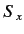given in Equation (427). We find that Equation (443) becomes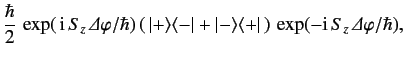(444)

or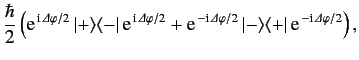(445)

which reduces to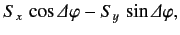(446)

where use has been made of Equations (427)-(429).

A second approach is to use the so called Baker-Hausdorff lemma. This takes the form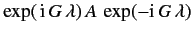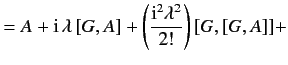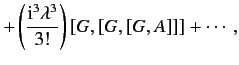(447)

where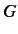is an Hermitian operator, and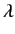a real parameter. The proof of this lemma is left as an exercise. Applying the Baker-Hausdorff lemma to Equation (443), we obtain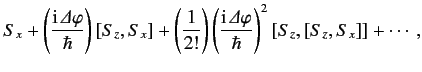(448)

which reduces to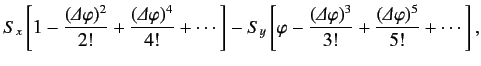(449)

or(450)

where use has been made of Equation (417). The second proof is more general than the first, because it only uses the fundamental commutation relation (417), and is, therefore, valid for systems with spin angular momentum higher than one-half.

For a spin one-half system, both methods imply that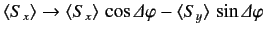(451)

under the action of the rotation operator (440). It is straightforward to show that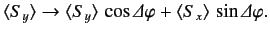(452)

Furthermore,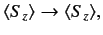(453)

because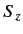commutes with the rotation operator. Equations (451)-(453) demonstrate that the operator (440) rotates the expectation value of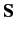by an angleabout the-axis. In fact, the expectation value of the spin operator behaves like a classical vector under rotation: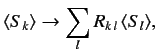(454)

where the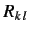are the elements of the conventional rotation matrix for the rotation in question. It is clear, from our second derivation of the result (451), that this property is not restricted to the spin operators of a spin one-half system. In fact, we have effectively demonstrated that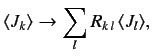(455)

where the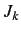are the generators of rotation, satisfying the fundamental commutation relation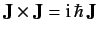, and the rotation operator about theth axis is written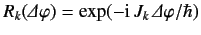.

Consider the effect of the rotation operator (440) on the state ket (439). It is easily seen that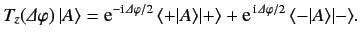(456)

Consider a rotation by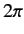radians. We find that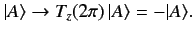(457)

Note that a ket rotated byradians differs from the original ket by a minus sign. In fact, a rotation by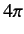radians is needed to transform a ket into itself. The minus sign does not affect the expectation value of, sinceis sandwiched between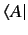and, both of which change sign. Nevertheless, the minus sign does give rise to observable consequences, as we shall see presently.Next: Magnetic Moments Up: Spin Angular Momentum Previous: Wavefunction of Spin One-Half
Richard Fitzpatrick 2013-04-08